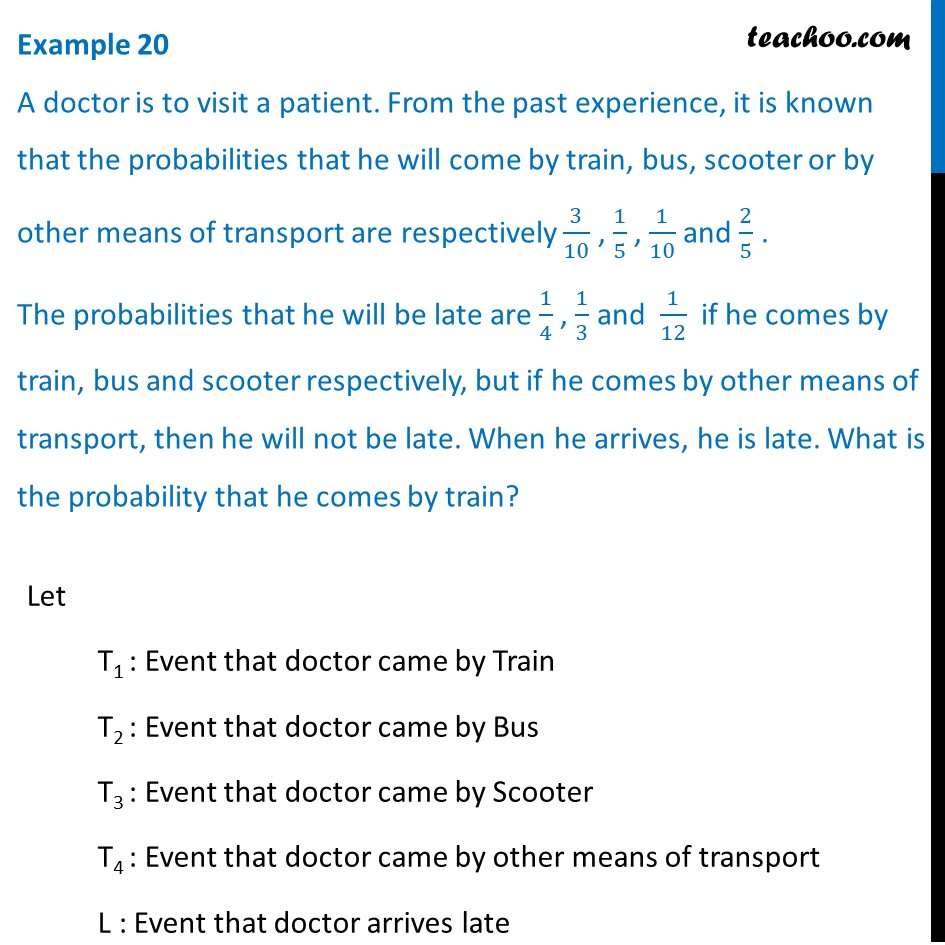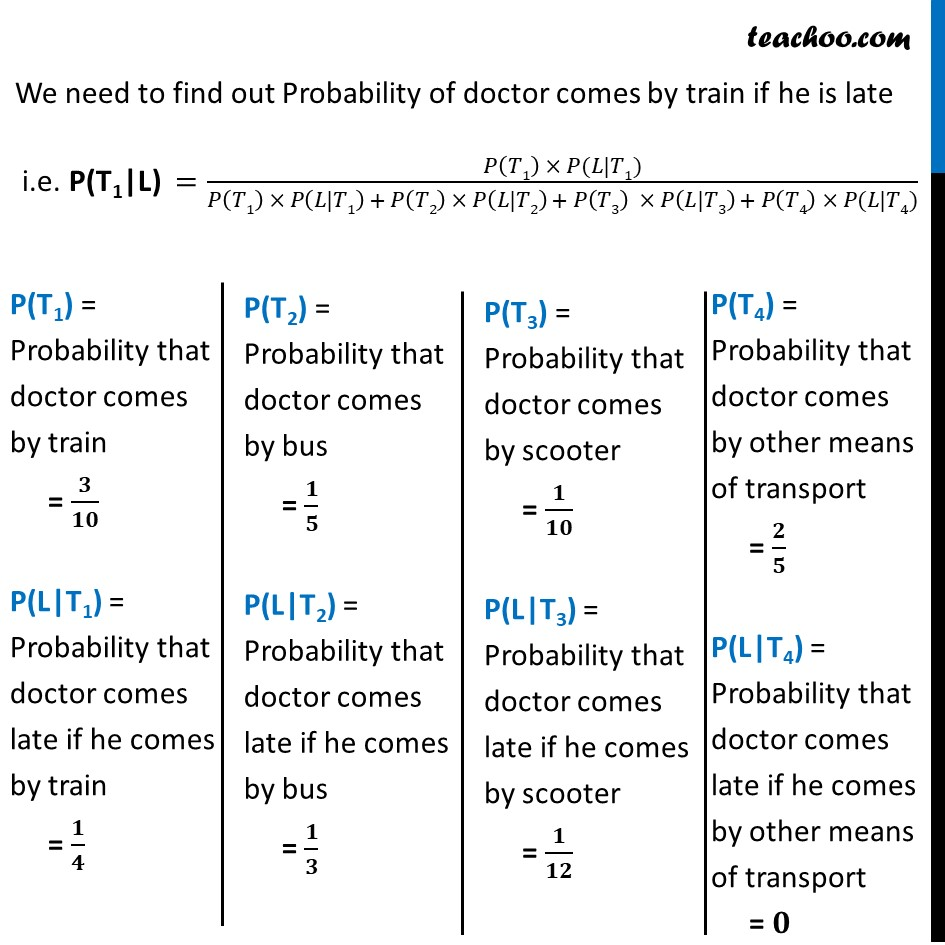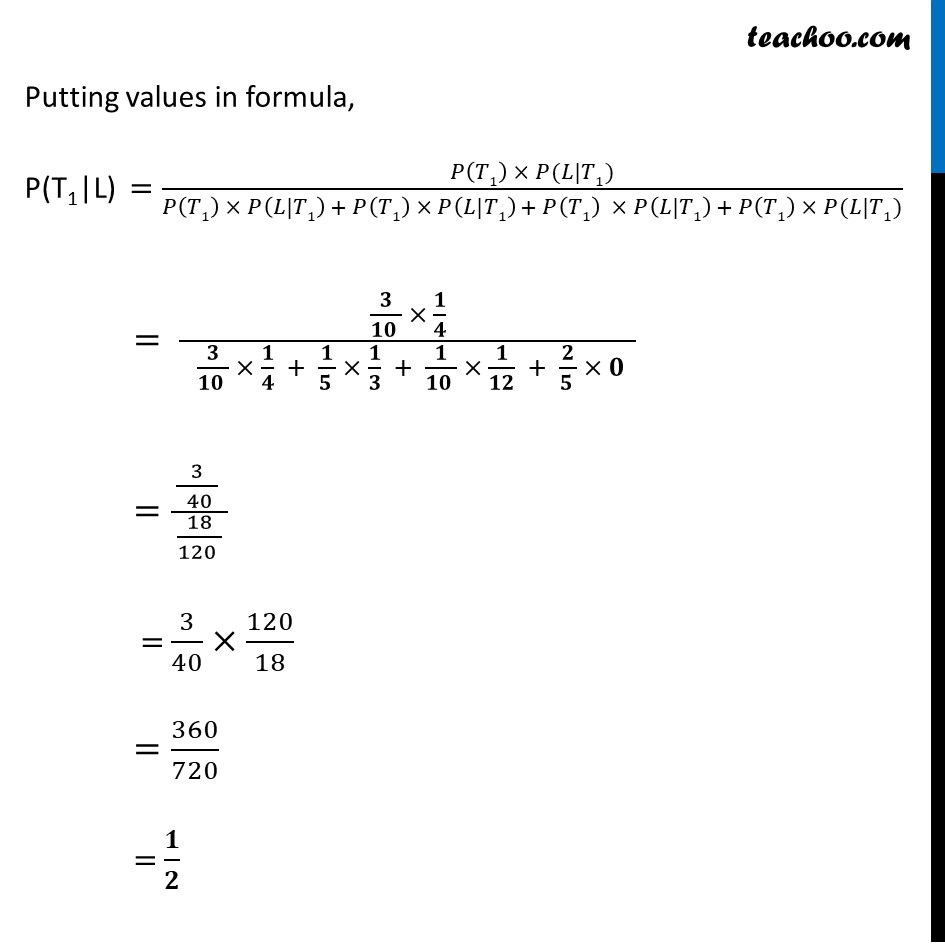Examples

Chapter 13 Class 12 Probability
Serial order wiseLearn in your speed, with individual attention - Teachoo Maths 1-on-1 Class

### Transcript

Example 20 A doctor is to visit a patient. From the past experience, it is known that the probabilities that he will come by train, bus, scooter or by other means of transport are respectively 3/10 , 1/5 , 1/10 and 2/5 . The probabilities that he will be late are 1/4 , 1/3 and 1/12 if he comes by train, bus and scooter respectively, but if he comes by other means of transport, then he will not be late. When he arrives, he is late. What is the probability that he comes by train?Let T1 : Event that doctor came by Train T2 : Event that doctor came by Bus T3 : Event that doctor came by Scooter T4 : Event that doctor came by other means of transport L : Event that doctor arrives late We need to find out Probability of doctor comes by train if he is late i.e. P(T1|L) =(𝑃(𝑇1) × 𝑃(𝐿|𝑇1))/(𝑃(𝑇1) × 𝑃(𝐿|𝑇1) + 𝑃(𝑇2) × 𝑃(𝐿|𝑇2) + 𝑃(𝑇3) × 𝑃(𝐿|𝑇3) + 𝑃(𝑇4) × 𝑃(𝐿|𝑇4)) P(T1) = Probability that doctor comes by train = 𝟑/𝟏𝟎 P(L|T1) = Probability that doctor comes late if he comes by train = 𝟏/𝟒 P(T2) = Probability that doctor comes by bus = 𝟏/𝟓 P(L|T2) = Probability that doctor comes late if he comes by bus = 𝟏/𝟑 P(T3) = Probability that doctor comes by scooter = 𝟏/𝟏𝟎 P(L|T3) = Probability that doctor comes late if he comes by scooter = 𝟏/𝟏𝟐 P(T4) = Probability that doctor comes by other means of transport = 𝟐/𝟓 P(L|T4) = Probability that doctor comes late if he comes by other means of transport = 𝟎 Putting values in formula, P(T1|L) =(𝑃(𝑇1) × 𝑃(𝐿|𝑇1))/(𝑃(𝑇1) × 𝑃(𝐿|𝑇1) + 𝑃(𝑇1) × 𝑃(𝐿|𝑇1) + 𝑃(𝑇1) × 𝑃(𝐿|𝑇1) + 𝑃(𝑇1) × 𝑃(𝐿|𝑇1)) = (𝟑/(𝟏𝟎 ) × 𝟏/𝟒)/( 𝟑/(𝟏𝟎 ) × 𝟏/𝟒 + 𝟏/(𝟓 ) × 𝟏/𝟑 + 𝟏/(𝟏𝟎 ) × 𝟏/𝟏𝟐 + 𝟐/(𝟓 ) × 𝟎 ) =(3/( 40 ) )/( 18/(120 ) ) = 3/40×120/18 =360/720 = 𝟏/𝟐